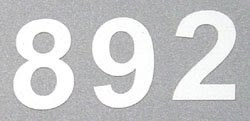## Tuesday, July 7, 2009

### 892

892 = 22 x 223.

892 is the smallest integer ratio of a 13-digit number to its product of digits.

892 is a number that cannot be written as a sum of three squares.

892 is a number such that the sum of its base 3 digits (1020001) equals the sum of its base 9 digits (1201).NGC 892 is a galaxy in the constellation Cetus.